# Acceleration

Describe how the cyclist's acceleration changes on individual sections (sections AB plane, BC turn, CD plane, DA turn), which describes the trajectory in the shape of an eight at a constant speed. The speed on the cyclist's tachometer is constant

a =  0 m/s2Did you find an error or inaccuracy? Feel free to write us. Thank you!Tips to related online calculators

#### You need to know the following knowledge to solve this word math problem:

We encourage you to watch this tutorial video on this math problem:

## Related math problems and questions:

• Tachometer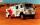Tatra's tachometer shows the initial state 886123 km this morning. Tatra today travels at an average speed of 44 km/h. Determine the function that describes the Tatra's tachometer depending on the time. What is the state of the tachometer after 4 hours?
• 2 cyclists and carOne cyclist rides at a constant speed over a bridge. It is 100 meters long. When he is 40 meters behind him, he meets an oncoming cyclist who is riding at the same speed. The car travels along the bridge in the same direction as the first cyclist at a spe
• The body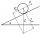The body slides down an inclined plane forming an angle α = π / 4 = 45° under the action of a horizontal plane under the effect of friction forces with acceleration a = 2.4 m/s ^ 2. At what angle β must the plane be inclined so that the body slides on it
• Ratio of squaresA circle is given in which a square is inscribed. The smaller square is inscribed in a circular arc formed by the side of the square and the arc of the circle. What is the ratio of the areas of the large and small squares?
• Angled cyclist turnThe cyclist passes through a curve with a radius of 20 m at 25 km/h. How much angle does it have to bend from the vertical inward to the turn?
• Circular railwayThe railway connects in a circular arc the points A, B, and C, whose distances are | AB | = 30 km, AC = 95 km, BC | = 70 km. How long will the track from A to C?
• Arc and segmentCalculate the length of circular arc l, area of the circular arc S1 and area of circular segment S2. Radius of the circle is 11 and corresponding angle is (2)/(12) π.
• Constant Angular Acceleration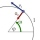The particle began to move from rest along a circle with a constant angular acceleration. After five cycles (n = 5), its angular velocity reached the value ω = 12 rad/s. Calculate the magnitude of the angular acceleration ε of this motion and the time int
• FlakesWe describe a circle of the square, and we describe a semicircle above each side of the square. This created 4 flakes. Which is bigger: the area of the central square, or the area of four flakes?
• Internal anglesThe ABCD is an isosceles trapezoid, which holds: |AB| = 2 |BC| = 2 |CD| = 2 |DA|: On its side BC is a K point such that |BK| = 2 |KC|, on its side CD is the point L such that |CL| = 2 |LD|, and on its side DA the point M is such that | DM | = 2 |MA|. Dete
• Uphill and downhillThe cyclist moves uphill at a constant speed of v1 = 10 km/h. When he reaches the top of the hill, he turns and passes the same track downhill at a speed of v2 = 40 km/h. What is the average speed of a cyclist?
• Circle arcCalculate the circular arc area in m2 where the diameter is 263 dm, and a central angle is 40°. Result round to three decimal places.
• Track arc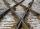Two straight tracks is in an angle 74°. They will join with circular arc with radius r=1127 m. How long will be arc connecting these lines (L)? How far is the center point of arc from track crossings (x)?
• A bridgeA bridge over a river has the shape of the arc with bases of the bridge at the river's edge. At the center of the river, the bridge is 10 feet above the water. At 27 feet from the edge of the river, the bridge is 9 feet above the water. How wide is the ri
• Chord BC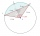A circle k has the center at the point S = [0; 0]. Point A = [40; 30] lies on the circle k. How long is the chord BC if the center P of this chord has the coordinates: [- 14; 0]?
• An accelerationThe car goes on a straight road at a speed of 72 km/h. At some point, the driver starts to brake and stops the car in 5 seconds. Find: (a) the acceleration during braking (b) the distance traveled during braking.
• Circular motionThe mass point regularly moves in a circle with radius r = 3.4 m angular velocity ω = 3.6 rad/s. Calculate the period, frequency, and centripetal acceleration of this movement.